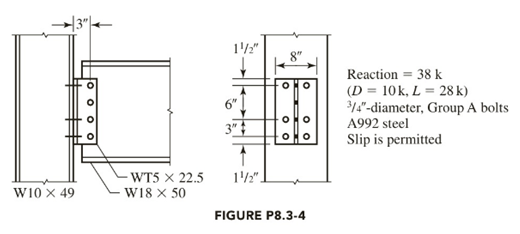Chapter 8, Problem 8.3.4P

Chapter
Section
Textbook Problem

Check the adequacy of the bolts. The given load is a service load.a. Use LRFD.b. Use ASD.To determine

(a)

The adequacy of the bolts using LRFD.

Explanation

Given:

The diameter of bolt is 34inch.

The live load was 28kips.

Concept Used:

Write the equation to calculate the nominal tensile stress modified to shear stress.

Fnt=1.3FntFntϕFnvfrvFnt     ...... (I)

Here, the nominal tensile stress modified to shear stress is Fnt, nominal tensile stress is Fnt, the load resistance factor is ϕ, the nominal shear strength is Fnv and the required shear stress is frv.

Write the equation to calculate the design tensile strength.

ϕRn=ϕFntAb ...... (II)

Here, the nominal bolt area is Ab and the design strength is ϕRn.

Calculation:

Write the equation to calculate the factored load for LRFD.

Pu=1.2(DL)+1.6(LL) ...... (III)

Here, the dead load is DL and the live load is LL.

Substitute 10kips for DL and 28kips for LL in Equation (III).

Pu=1.2(10kips)+1.6(28kips)=12kips+44.8kips=56.8kips

Write the equation to calculate the nominal shear capacity of bolt.

Rnv=nFnvAb     ...... (IV)

Here, the nominal shear capacity is Rnv, the nominal shear strength is Fnv, the number of shear failure is n and the nominal bolt area is Ab.

Calculate the nominal bolt area.

Ab=π4d2 ...... (V)

Here, the diameter of bolt is d.

Substitute 34inch for d in Equation (V).

Ab=π4(34in)2=0.4417in2

For A992 steel the value of Fnv is 48ksi.

Calculate the nominal shear capacity of bolt.

Substitute 48ksi for Fnv, 0.4417in2 for Ab and 1 for n in Equation (IV).

Rnv=1×48ksi×0.4417in2=21.20kips

Write the equation to calculate the bearing deformation strength of bolt.

Rbd=2.4dtFu ...... (VI)

Here, the bearing deformation strength is Rbd, the thickness of the connected part is t and the ultimate tensile stress of gusset plate is Fu.

For A992 steel the value of Fu is 65ksi.

Calculate the bearing deformation strength of bolt.

Substitute 34inch for d, 65ksi for Fu and 0.375inch for t in Equation (VI).

Rbd=2.4×(34in)×(0.375in)×65ksi=0.675in2×65kips/in2=43.875kips

Write the equation to calculate the tear-out strength of inner bolt.

Rti=1.2lctFu ...... (VII)

Here, the tear-out strength of inner bolt is Rti and the clear distance, in the direction parallel to the applied load from the edge of bolt hole to the edge of the adjacent hole or to the edge of the material is lc.

Write the equation to calculate the clear distance for edge bolt.

lc=leh2 ...... (VIII)

Here, the bolt edge distance is le and the bolt hole diameter is h.

Write the equation to calculate the hole diameter.

h=d+116in ...... (IX)

Substitute 34inch for d in Equation (IX).

h=34in+116in=0.75in+0.0625in=0.8125in

Calculate the clear distance for edge bolt.

Substitute 0.8125in for h and 2in for le in Equation (VIII).

lc=2in0.8125in2=2in0.4062in=1.5938in

Calculate the tear-out strength of inner bolt.

Substitute 1.5938in for lc, 65ksi for Fu and 0.375inch for t in Equation (VII).

Rti=1.2×1.5938in×0.375in×65ksi=0.7172in2×65kips/in2=46

To determine

(b)

The adequacy of the bolts using ASD.

Still sussing out bartleby?

Check out a sample textbook solution.

See a sample solution

The Solution to Your Study Problems

Bartleby provides explanations to thousands of textbook problems written by our experts, many with advanced degrees!

Get Started

Find more solutions based on key concepts